#### Number of problems found: 156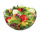We need two tenths kg of a carrot, one tenth of peas and three tenths of of tomatoes to make salad. Express the fraction of the weight of the vegetables to be salad. Convert the result to grams.
• Evaluate expressionEvaluate expression using BODMAS rule: 1 1/4+1 1/5÷3/5-5/8
• Series and sequencesFind a fraction equivalent to the recurring decimal? 0.435643564356
• Two numbersThe sum of two numbers is 1. Identify this two numbers if you know that the half of first is equal to the third of second number.
• Master and apprentice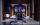Master painted the roof in 3 hours and apprentice for 4 hours. How many of roof they painted in hour and how many in three quarters of an hour?To three-eighths of one third, we add five quarters of one half and multiply the sum by four. How much will we get?
• Roses and tulipsAt the florist are 50 tulips and 5 times fewer roses. How many flowers are in the flower shop?
• Pupils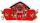Two classes want to collect money. Boys are four-seventh pupils. In time did not pay a quarter of the boys and a sixth of girls, which mean 12 sinners. How many pupils attend these two classes?
• Conversion of units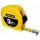Complete the following length data
• Stock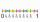Enterprise sold 7/12 of their products to foreign markets and 2/5 of the remainder sold at home. How many % of the products is still in stock?
• CupcakesIn a bowl was some cupcakes. Janka ate one third and Danka ate one quarter of cupcakes. a) How many of cookies ate together? b) How many cookies remain in a bowl? Write the results as a decimal number and in notepad also as a fraction.
• Decimal to fractionWrite decimal number 8.638333333 as a fraction A/B in the basic form. Given decimal has infinite repeating figures.
• Playing Cards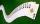Kara has 2 times more cards than Dana, Dana has 4× less than Mary. Together they have 728 cards. How many cards has each of them?
• EvaluateThe division of numbers 6 and 3 increase by-product of the numbers 115 and 0.1
• FractionFraction frac(0, overline(38))(0,38) write as fraction a/b, a, b is integers numerator/denominator.
• DiophantusWe know little about this Greek mathematician from Alexandria, except that he lived around 3rd century A. D. Thanks to an admirer of his, who described his life through an algebraic riddle, we know at least something about his life. Diophantus's youth las

Do you have an exciting math question or word problem that you can't solve? Ask a question or post a math problem, and we can try to solve it.

We will send a solution to your e-mail address. Solved examples are also published here. Please enter the e-mail correctly and check whether you don't have a full mailbox.

Need help to calculate sum, simplify or multiply fractions? Try our fraction calculator. Fraction Word Problems. Addition Problems.